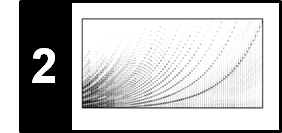# Digital Signals: Sampling

Analog signals have a continuous range of values in both time and amplitude, which generally leads to an infinite number of values. Since a computer can only store and process a finite number of values, one has to convert the waveform into some discrete representation—a process commonly referred to as digitization. The most common approach for digitizing audio signals consists of two steps called sampling and quantization. In this notebook, following Section 2.2.2.1 of [Müller, FMP, Springer 2015], we explain the concept of sampling in more detail.

## Sampling¶

In signal processing, the term sampling refers to the process of reducing a continuous-time (CT) signal to a discrete-time (DT) signal, which is defined only on a discrete subset of the time axis. By means of a suitable encoding, one often assumes that this discrete set is a subset $I$ of the set $\mathbb{Z}$ of integers. Then a DT-signal is defined to be a function $x\colon I\to\mathbb{R}$, where the domain $I$ corresponds to points in time. Since one can extend any DT-signal from the domain $I$ to the domain $\mathbb{Z}$ simply by setting all values to zeros for points in $\mathbb{Z}\setminus I$, we may assume $I=\mathbb{Z}$.

The most common sampling procedure to transform a CT-signal $f\colon\mathbb{R}\to\mathbb{R}$ into a DT-signal $x\colon\mathbb{Z}\to\mathbb{R}$ is known as equidistant sampling. Fixing a positive real number $T>0$, the DT-signal $x$ is obtained by setting

\begin{equation} x(n):= f(n \cdot T) \end{equation}

for $n\in\mathbb{Z}$. The value $x(n)$ is called the sample taken at time $t=n\cdot T$ of the original analog signal $f$. In short, this procedure is also called $T$-sampling. The number $T$ is referred to as the sampling period and the inverse $F_\mathrm{s}:=1/T$ as the sampling rate. The sampling rate specifies the number of samples per second and is measured in Hertz (Hz).

In the following code cell, we start with a CT-signal $f$ that is defined via linear interpolation of a DT-signal sampled at a high sampling rate. In the plot, this CT-signal is shown as black curve. Applying equidistant sampling, we obtain the DT-signal $x$ visualized as the red stem plot.

In :
import numpy as np
from scipy.interpolate import interp1d
from matplotlib import pyplot as plt
%matplotlib inline

def generate_function(Fs, dur=1):
"""Generate example function

Notebook: C2/C2S2_DigitalSignalSampling.ipynb

Args:
Fs (scalar): Sampling rate
dur (float): Duration (in seconds) of signal to be generated (Default value = 1)

Returns:
x (np.ndarray): Signal
t (np.ndarray): Time axis (in seconds)
"""
N = int(Fs * dur)
t = np.arange(N) / Fs
x = 1 * np.sin(2 * np.pi * (2 * t - 0))
x += 0.5 * np.sin(2 * np.pi * (6 * t - 0.1))
x += 0.1 * np.sin(2 * np.pi * (20 * t - 0.2))
return x, t

def sampling_equidistant(x_1, t_1, Fs_2, dur=None):
"""Equidistant sampling of interpolated signal

Notebook: C2/C2S2_DigitalSignalSampling.ipynb

Args:
x_1 (np.ndarray): Signal to be interpolated and sampled
t_1 (np.ndarray): Time axis (in seconds) of x_1
Fs_2 (scalar): Sampling rate used for equidistant sampling
dur (float): Duration (in seconds) of sampled signal (Default value = None)

Returns:
x (np.ndarray): Sampled signal
t (np.ndarray): Time axis (in seconds) of sampled signal
"""
if dur is None:
dur = len(t_1) * t_1
N = int(Fs_2 * dur)
t_2 = np.arange(N) / Fs_2
x_2 = interp1d(t_1, x_1, kind='linear', fill_value='extrapolate')(t_2)
return x_2, t_2

Fs_1 = 100
x_1, t_1 = generate_function(Fs=Fs_1, dur=2)

Fs_2 = 20
x_2, t_2 = sampling_equidistant(x_1, t_1, Fs_2)

plt.figure(figsize=(8, 2.2))
plt.plot(t_1, x_1, 'k')
plt.title('Original CT-signal')
plt.xlabel('Time (seconds)')
plt.ylim([-1.5, 1.5])
plt.xlim([t_1, t_1[-1]])
plt.tight_layout()

plt.figure(figsize=(8, 2.2))
plt.stem(t_2, x_2, linefmt='r', markerfmt='ro', basefmt='None', use_line_collection=True)
plt.plot(t_1, x_1, 'k', linewidth=1, linestyle='dotted')
plt.title(r'Sampling rate $F_\mathrm{s} = %.0f$'%Fs_2)
plt.xlabel('Time (seconds)')
plt.ylim([-1.5, 1.5])
plt.xlim([t_1, t_1[-1]])
plt.tight_layout()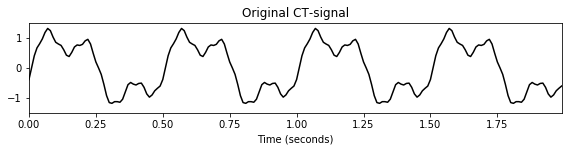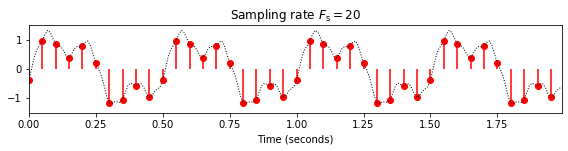## Aliasing¶

In general, sampling is a lossy operation in the sense that information is lost in this process and that the original CT-signal cannot be recovered from its sampled version. Only if the CT-signal has additional properties in terms of its frequency spectrum (it needs to be bandlimited), a perfect reconstruction is possible. This is the assertion of the famous sampling theorem, which we explain in more detail at the end of this notebook. The sampling theorem also shows how the original CT-signal can be reconstructed by superimposing suitably shifted $\mathrm{sinc}$-functions weighted by the samples of the DT-signal.

Without additional properties, sampling may cause an effect known as aliasing where certain frequency components of the signal become indistinguishable. This effect is illustrated by the following figure. Using a high sampling rate, the original CT-signal can be reconstructed with high accuracy. However, when decreasing the sampling rate, the higher-frequency components are not captured well and only a coarse approximation of the original signal remains. This phenomenon is demonstrated by the following example.

In :
def reconstruction_sinc(x, t, t_sinc):
"""Reconstruction from sampled signal using sinc-functions

Notebook: C2/C2S2_DigitalSignalSampling.ipynb

Args:
x (np.ndarray): Sampled signal
t (np.ndarray): Equidistant discrete time axis (in seconds) of x
t_sinc (np.ndarray): Equidistant discrete time axis (in seconds) of signal to be reconstructed

Returns:
x_sinc (np.ndarray): Reconstructed signal having time axis t_sinc
"""
Fs = 1 / t
x_sinc = np.zeros(len(t_sinc))
for n in range(0, len(t)):
x_sinc += x[n] * np.sinc(Fs * t_sinc - n)
return x_sinc

def plot_signal_reconstructed(t_1, x_1, t_2, x_2, t_sinc, x_sinc):
plt.figure(figsize=(8, 2.2))
plt.plot(t_1, x_1, 'k', linewidth=1, linestyle='dotted', label='Orignal signal')
plt.stem(t_2, x_2, linefmt='r:', markerfmt='r.', basefmt='None', label='Samples', use_line_collection=True)
plt.plot(t_1, x_sinc, 'b', label='Reconstructed signal')
plt.title(r'Sampling rate $F_\mathrm{s} = %.0f$'%(1/t_2))
plt.xlabel('Time (seconds)')
plt.ylim([-1.5, 1.5])
plt.xlim([t_1, t_1[-1]])
plt.legend(loc='upper right', framealpha=1)
plt.tight_layout()
plt.show()

Fs_2 = 40
x_2, t_2 = sampling_equidistant(x_1, t_1, Fs_2)
t_sinc = t_1
x_sinc = reconstruction_sinc(x_2, t_2, t_sinc)
plot_signal_reconstructed(t_1, x_1, t_2, x_2, t_sinc, x_sinc);

Fs_2 = 20
x_2, t_2 = sampling_equidistant(x_1, t_1, Fs_2)
t_sinc = t_1
x_sinc = reconstruction_sinc(x_2, t_2, t_sinc)
plot_signal_reconstructed(t_1, x_1, t_2, x_2, t_sinc, x_sinc);

Fs_2 = 10
x_2, t_2 = sampling_equidistant(x_1, t_1, Fs_2)
t_sinc = t_1
x_sinc = reconstruction_sinc(x_2, t_2, t_sinc)
plot_signal_reconstructed(t_1, x_1, t_2, x_2, t_sinc, x_sinc);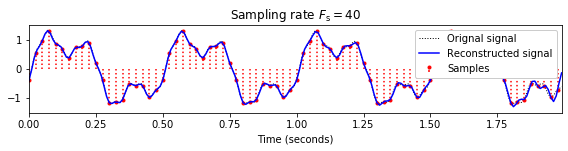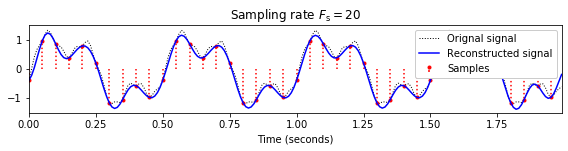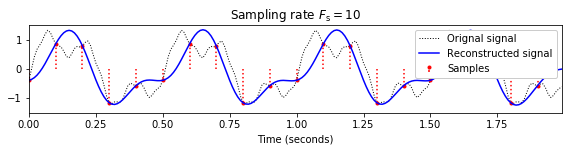Aliasing effects occur also in other areas such as the visual domain. One famous example is the wagon-wheel effect, where a wheel appears to rotate at a slower speed or even backwards when the speed is actually increasing.

In :
import IPython.display as ipd


The next example indicates how aliasing effects the sound quality. We start with a music signal at a high sampling rate ($F_\mathrm{s}=8192$ Hz), which is then successively reduced by a factor of two.

In :
import os
import IPython.display as ipd
import librosa
import scipy.signal

path_filename_wav = os.path.join('..', 'data', 'C2', 'FMP_C2_Sampling_C-major-scale.wav')
Fs_orig = Fs
len_orig = len(x)
for i in range(5):
print('Sampling rate Fs = %s; Number of samples = %s' % (Fs, len(x)), flush=True)
# ipd.display(ipd.Audio(data=x, rate=Fs))
# Some web browsers do not support arbitray sample rates.
# Therefore, the previous output may work for some web rowers.
# This is a work around:
x_play = scipy.signal.resample(x, len_orig)
ipd.display(ipd.Audio(data=x_play, rate=Fs_orig))
Fs = Fs // 2
x = x[::2]

Sampling rate Fs = 8000; Number of samples = 26496

Sampling rate Fs = 4000; Number of samples = 13248

Sampling rate Fs = 2000; Number of samples = 6624

Sampling rate Fs = 1000; Number of samples = 3312

Sampling rate Fs = 500; Number of samples = 1656


## Sampling Theorem¶

The sampling theorem, which is often associated with the names Harry Nyquist and Claude Shannon, states that a continuous-time (CT) signal that is bandlimited can be reconstructed perfectly under certain conditions. More precisely, a CT-signal $f\in L^2(\mathbb{R})$ is called $\Omega$-bandlimited if the Fourier transform $\hat{f}$ vanishes for $|\omega|>\Omega$ (i.e., $\hat{f}(\omega) = 0$ for $|\omega|>\Omega$). Let $f\in L^2(\mathbb{R})$ be an $\Omega$-bandlimited function and let $x$ be the $T$-sampled version of $f$ with $T:=1/(2\Omega)$ (i.e., $x(n)=f(nT)$, $n\in\mathbb{Z}$). Then $f$ can be reconstructed from $x$ by

$$f(t)=\sum_{n\in\mathbb{Z}}x(n)\mathrm{sinc}\left(\frac{t-nT}{T}\right) =\sum_{n\in\mathbb{Z}}f\left(\frac{n}{2\Omega}\right) \mathrm{sinc}\left(2\Omega t-n\right),$$

where the $\mathrm{sinc}$-function is defined as

\begin{equation} \mathrm{sinc}(t):=\left\{\begin{array}{ll} \frac{\sin \pi t}{\pi t},&\mbox{ if $t\not= 0$,}\\ 1,&\mbox{ if $t= 0$.} \end{array}\right. \end{equation}

In other words, the CT-signal $f$ can be perfectly reconstructed from the DT-signal obtained by equidistant sampling if the bandlimit is no greater than half the sampling rate. This reconstruction based on the $\mathrm{sinc}$-function has been used in the function reconstruction_sinc.

Acknowledgment: This notebook was created by Meinard Müller.